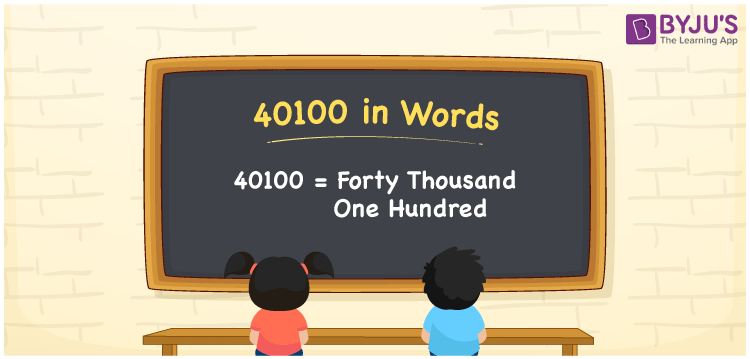# 40100 in Words

40100 in words is forty thousand one hundred. If you purchased a two-wheeler that costs Rs. 40100, it can be written as “I purchased a two-wheeler that costs Rs. Forty thousand one hundred. In this article, let us discuss how to write the number 40100 in words using the place value system in detail.

 40100 in Words: Forty Thousand One Hundred. Forty Thousand One Hundred in Numerical Form: 40100.

## 40100 in English Words## How to Write 40100 in Words?

Learn the place values of 40100 upto five digits.

 Ten-thousands Thousands Hundreds Tens Ones 4 0 1 0 0

The expanded form of 40100 is as follows:

= 4 × Ten thousand + 0 × Thousand + 1 × Hundred + 0 × Ten + 0 × One

= 4 × 10000 + 0 × 1000 + 1 × 100 + 0 × 10 + 0 × 1

= 40000 + 100

= 40100

= Forty thousand one hundred

Hence, 40100 in words is forty thousand one hundred.

40100 in words – Forty thousand one hundred

Is 40100 an odd number? – No

Is 40100 an even number? – Yes

Is 40100 a perfect square number? – No

Is 40100 a perfect cube number? – No

Is 40100 a prime number? – No

Is 40100 a composite number? – Yes

## Frequently Asked Questions on 40100 in Words

Q1

### Write 40100 in English words.

40100 in words is forty thousand one hundred.

Q2

### Simplify 40000 + 100, and express it in words.

Simplifying 40000 + 100, we get 40100. Hence, 40100 in words is forty thousand one hundred.

Q3

### Convert forty thousand one hundred into numbers.

Forty thousand one hundred in numbers is 40100.